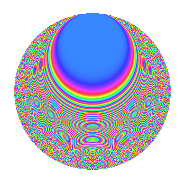Properties

 Label 6019.2.a.eLevel 6019 Weight 2 Character orbit 6019.a Self dual Yes Analytic conductor 48.062 Analytic rank 0 Dimension 130 CM No

Related objects

Newspace parameters

 Level: $$N$$ = $$6019 = 13 \cdot 463$$ Weight: $$k$$ = $$2$$ Character orbit: $$[\chi]$$ = 6019.a (trivial)

Newform invariants

 Self dual: Yes Analytic conductor: $$48.0619569766$$ Analytic rank: $$0$$ Dimension: $$130$$ Fricke sign: $$-1$$ Sato-Tate group: $\mathrm{SU}(2)$

$q$-expansion

The dimension is sufficiently large that we do not compute an algebraic $$q$$-expansion, but we have computed the trace expansion.

 $$\operatorname{Tr}(f)(q) =$$ $$130q$$ $$\mathstrut +\mathstrut 10q^{2}$$ $$\mathstrut +\mathstrut 11q^{3}$$ $$\mathstrut +\mathstrut 146q^{4}$$ $$\mathstrut +\mathstrut 40q^{5}$$ $$\mathstrut +\mathstrut 4q^{6}$$ $$\mathstrut +\mathstrut 8q^{7}$$ $$\mathstrut +\mathstrut 24q^{8}$$ $$\mathstrut +\mathstrut 181q^{9}$$ $$\mathstrut +\mathstrut O(q^{10})$$ $$\operatorname{Tr}(f)(q) =$$ $$130q$$ $$\mathstrut +\mathstrut 10q^{2}$$ $$\mathstrut +\mathstrut 11q^{3}$$ $$\mathstrut +\mathstrut 146q^{4}$$ $$\mathstrut +\mathstrut 40q^{5}$$ $$\mathstrut +\mathstrut 4q^{6}$$ $$\mathstrut +\mathstrut 8q^{7}$$ $$\mathstrut +\mathstrut 24q^{8}$$ $$\mathstrut +\mathstrut 181q^{9}$$ $$\mathstrut +\mathstrut 5q^{10}$$ $$\mathstrut +\mathstrut 43q^{11}$$ $$\mathstrut +\mathstrut 28q^{12}$$ $$\mathstrut +\mathstrut 130q^{13}$$ $$\mathstrut +\mathstrut 47q^{14}$$ $$\mathstrut +\mathstrut 29q^{15}$$ $$\mathstrut +\mathstrut 170q^{16}$$ $$\mathstrut +\mathstrut 85q^{17}$$ $$\mathstrut +\mathstrut 20q^{18}$$ $$\mathstrut +\mathstrut 3q^{19}$$ $$\mathstrut +\mathstrut 73q^{20}$$ $$\mathstrut +\mathstrut 62q^{21}$$ $$\mathstrut +\mathstrut 12q^{22}$$ $$\mathstrut +\mathstrut 62q^{23}$$ $$\mathstrut -\mathstrut 5q^{24}$$ $$\mathstrut +\mathstrut 178q^{25}$$ $$\mathstrut +\mathstrut 10q^{26}$$ $$\mathstrut +\mathstrut 35q^{27}$$ $$\mathstrut -\mathstrut q^{28}$$ $$\mathstrut +\mathstrut 134q^{29}$$ $$\mathstrut +\mathstrut 24q^{30}$$ $$\mathstrut +\mathstrut 14q^{31}$$ $$\mathstrut +\mathstrut 48q^{32}$$ $$\mathstrut +\mathstrut 4q^{33}$$ $$\mathstrut +\mathstrut 4q^{34}$$ $$\mathstrut +\mathstrut 50q^{35}$$ $$\mathstrut +\mathstrut 244q^{36}$$ $$\mathstrut +\mathstrut 32q^{37}$$ $$\mathstrut +\mathstrut 76q^{38}$$ $$\mathstrut +\mathstrut 11q^{39}$$ $$\mathstrut -\mathstrut 6q^{40}$$ $$\mathstrut +\mathstrut 48q^{41}$$ $$\mathstrut +\mathstrut 9q^{42}$$ $$\mathstrut +\mathstrut 34q^{43}$$ $$\mathstrut +\mathstrut 123q^{44}$$ $$\mathstrut +\mathstrut 115q^{45}$$ $$\mathstrut +\mathstrut 5q^{46}$$ $$\mathstrut +\mathstrut 25q^{47}$$ $$\mathstrut +\mathstrut 35q^{48}$$ $$\mathstrut +\mathstrut 210q^{49}$$ $$\mathstrut +\mathstrut 24q^{50}$$ $$\mathstrut +\mathstrut 20q^{51}$$ $$\mathstrut +\mathstrut 146q^{52}$$ $$\mathstrut +\mathstrut 193q^{53}$$ $$\mathstrut -\mathstrut 39q^{54}$$ $$\mathstrut +\mathstrut 32q^{55}$$ $$\mathstrut +\mathstrut 122q^{56}$$ $$\mathstrut +\mathstrut 7q^{57}$$ $$\mathstrut -\mathstrut 4q^{58}$$ $$\mathstrut +\mathstrut 50q^{59}$$ $$\mathstrut +\mathstrut 42q^{60}$$ $$\mathstrut +\mathstrut 57q^{61}$$ $$\mathstrut +\mathstrut 51q^{62}$$ $$\mathstrut +\mathstrut 8q^{63}$$ $$\mathstrut +\mathstrut 172q^{64}$$ $$\mathstrut +\mathstrut 40q^{65}$$ $$\mathstrut -\mathstrut 4q^{66}$$ $$\mathstrut +\mathstrut 21q^{67}$$ $$\mathstrut +\mathstrut 132q^{68}$$ $$\mathstrut +\mathstrut 92q^{69}$$ $$\mathstrut -\mathstrut 46q^{70}$$ $$\mathstrut +\mathstrut 58q^{71}$$ $$\mathstrut -\mathstrut 26q^{72}$$ $$\mathstrut +\mathstrut 15q^{73}$$ $$\mathstrut +\mathstrut 120q^{74}$$ $$\mathstrut +\mathstrut 23q^{75}$$ $$\mathstrut -\mathstrut 65q^{76}$$ $$\mathstrut +\mathstrut 192q^{77}$$ $$\mathstrut +\mathstrut 4q^{78}$$ $$\mathstrut +\mathstrut 32q^{79}$$ $$\mathstrut +\mathstrut 66q^{80}$$ $$\mathstrut +\mathstrut 326q^{81}$$ $$\mathstrut +\mathstrut 11q^{82}$$ $$\mathstrut +\mathstrut 33q^{83}$$ $$\mathstrut +\mathstrut 5q^{84}$$ $$\mathstrut +\mathstrut 43q^{85}$$ $$\mathstrut +\mathstrut 105q^{86}$$ $$\mathstrut +\mathstrut 31q^{87}$$ $$\mathstrut -\mathstrut 17q^{88}$$ $$\mathstrut +\mathstrut 84q^{89}$$ $$\mathstrut -\mathstrut 73q^{90}$$ $$\mathstrut +\mathstrut 8q^{91}$$ $$\mathstrut +\mathstrut 161q^{92}$$ $$\mathstrut +\mathstrut 52q^{93}$$ $$\mathstrut +\mathstrut 4q^{94}$$ $$\mathstrut +\mathstrut 59q^{95}$$ $$\mathstrut -\mathstrut 77q^{96}$$ $$\mathstrut +\mathstrut 9q^{97}$$ $$\mathstrut -\mathstrut 61q^{98}$$ $$\mathstrut +\mathstrut 100q^{99}$$ $$\mathstrut +\mathstrut O(q^{100})$$

Embeddings

For each embedding $$\iota_m$$ of the coefficient field, the values $$\iota_m(a_n)$$ are shown below.

For more information on an embedded modular form you can click on its label.

Label $$a_{2}$$ $$a_{3}$$ $$a_{4}$$ $$a_{5}$$ $$a_{6}$$ $$a_{7}$$ $$a_{8}$$ $$a_{9}$$ $$a_{10}$$
1.1 −2.80453 3.24972 5.86540 2.53460 −9.11395 2.92678 −10.8406 7.56069 −7.10835
1.2 −2.76072 1.85217 5.62158 −1.74367 −5.11334 −4.49860 −9.99819 0.430551 4.81380
1.3 −2.70229 −1.73872 5.30239 −0.220244 4.69853 −4.26508 −8.92402 0.0231491 0.595165
1.4 −2.65690 −1.17656 5.05912 −3.97344 3.12601 −1.98444 −8.12779 −1.61571 10.5570
1.5 −2.57909 0.0546080 4.65172 2.10708 −0.140839 −1.58025 −6.83904 −2.99702 −5.43435
1.6 −2.55222 −2.23917 4.51381 1.89858 5.71484 −3.17598 −6.41579 2.01387 −4.84558
1.7 −2.52323 3.28941 4.36670 3.66616 −8.29994 −5.12423 −5.97175 7.82019 −9.25058
1.8 −2.52101 −1.65021 4.35552 2.33988 4.16020 4.41588 −5.93829 −0.276814 −5.89887
1.9 −2.51967 −3.32656 4.34873 −0.493062 8.38182 −0.741150 −5.91802 8.06597 1.24235
1.10 −2.50771 −1.46096 4.28860 1.53494 3.66367 0.422474 −5.73914 −0.865591 −3.84918
1.11 −2.49834 2.94692 4.24169 −1.72770 −7.36239 −2.26517 −5.60049 5.68431 4.31639
1.12 −2.49278 −3.38832 4.21397 3.98474 8.44636 1.44276 −5.51896 8.48073 −9.93310
1.13 −2.48853 0.814378 4.19279 −1.02272 −2.02661 0.518159 −5.45682 −2.33679 2.54508
1.14 −2.40942 1.96845 3.80532 3.79888 −4.74282 2.98166 −4.34977 0.874791 −9.15310
1.15 −2.39514 1.83196 3.73671 3.10935 −4.38781 4.25584 −4.15967 0.356083 −7.44733
1.16 −2.34374 2.49578 3.49312 −2.30500 −5.84945 0.790647 −3.49949 3.22890 5.40232
1.17 −2.33535 −1.90450 3.45386 −1.81837 4.44767 2.51906 −3.39528 0.627108 4.24653
1.18 −2.28421 0.124993 3.21761 −2.44620 −0.285511 −2.39008 −2.78127 −2.98438 5.58762
1.19 −2.17988 −0.326610 2.75186 0.291618 0.711970 2.70764 −1.63895 −2.89333 −0.635691
1.20 −2.07170 0.913939 2.29195 −0.284252 −1.89341 −4.46498 −0.604826 −2.16472 0.588884
See next 80 embeddings (of 130 total)
 $$n$$: e.g. 2-40 or 990-1000 Embeddings: e.g. 1-3 or 1.130 Significant digits: Format: Complex embeddings Normalized embeddings Satake parameters Satake angles

Inner twists

This newform does not have CM; other inner twists have not been computed.

Atkin-Lehner signs

$$p$$ Sign
$$13$$ $$-1$$
$$463$$ $$1$$

Hecke kernels

This newform can be constructed as the kernel of the linear operator $$T_{2}^{130} - \cdots$$ acting on $$S_{2}^{\mathrm{new}}(\Gamma_0(6019))$$.